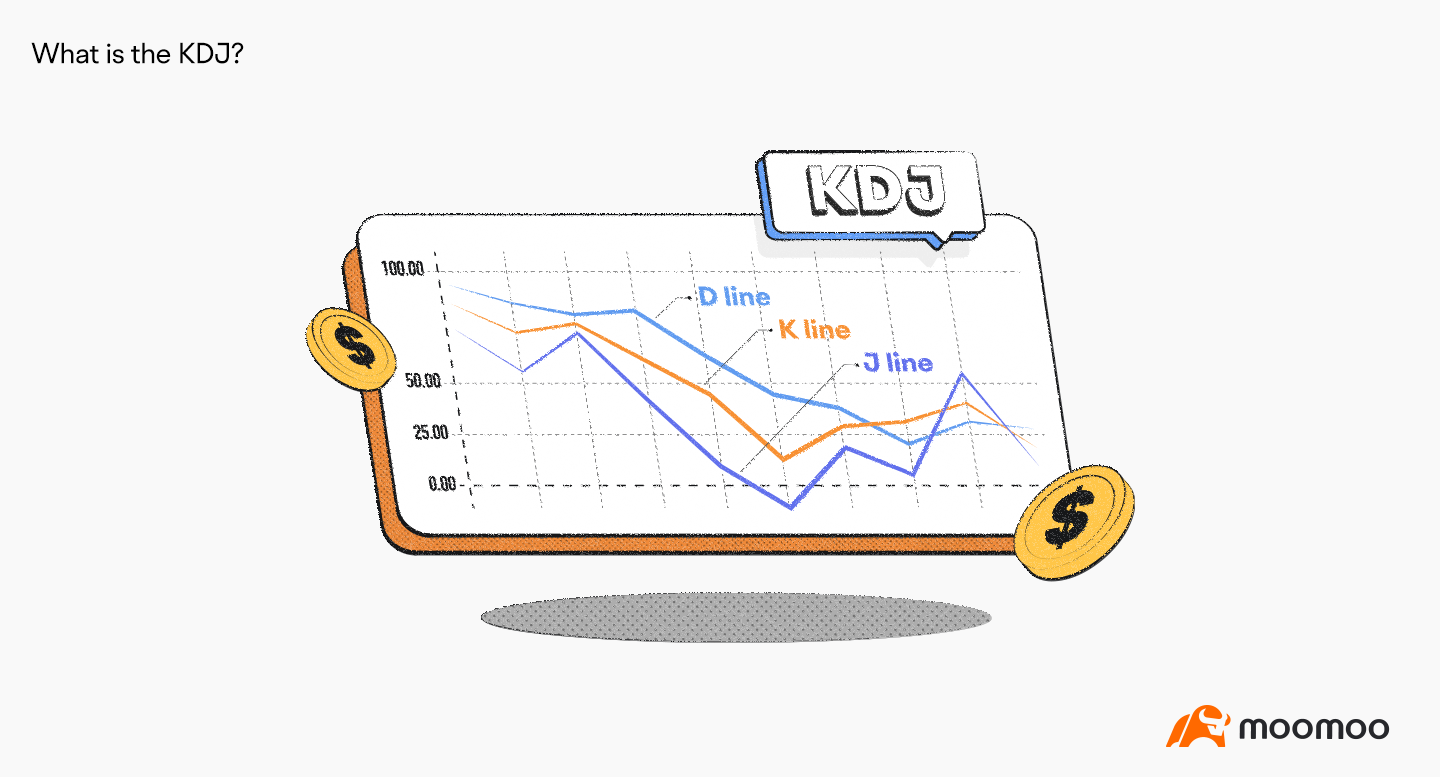• English
• 中文繁体
• 中文简体
Hi,
moomoo ID:NaN
Log Out

# What Is The KDJ?

Views 662022.08.03### Key takeaways

The KDJ, also known as a stochastic indicator, is a technical indicator widely used in the futures and stock markets for short-term trend analysis.

The KDJ reflects the strength of price movements, overbought and oversold signals, and trading signals.

The KDJ may not work in a highly volatile market.

### Understanding the KDJ

The KDJ, also known as a stochastic index, was first used in the analysis of the futures market and is widely used in the short-term trend analysis of the stock market. The KDJ is calculated based on the highest price, the lowest price, and the closing price. It uses the realism of price fluctuations to reflect the strength of price fluctuations, overbought and oversold, and gives trading signals before prices rise or fall. The KDJ is plotted as three lines.

（Images provided are not current and any securities are shown for illustrative purposes only.）

### Calculation of KDJ

The KDJ is used to obtain the random stochastic value (RSV) by calculating the proportional relationship between the highest, lowest, and closing prices that have occurred in a specific period and then smoothing the corresponding data to obtain a series of index values that are plotted to determine the movement of securities. The specific calculation is below.

First, calculate the RSV value of the period, then calculate the K value, D value, and J value. The KDJ can characterize short- and medium-term market fluctuations by setting different durations of time periods.

Taking the calculation of the daily KDJ value as an example, the calculation formula is:

The N day RSV=(Cn-Ln) ÷(Hn-Ln) ×100.

In the formula, Cn is the closing price on the nth day; Ln is the lowest price in n days; Hn is the highest price in n days. The RSV value always fluctuates between 1 and 100.

Second, calculate the K and D values:

The day K value = 2 / 3 × the previous day K value + 1/3 × the day RSV

The Day D value = 2 / 3 × the previous day D value + 1/3 × the day K value

The J value = 3 × the day K value - 2 × the day D value

If there is no K value and D value on the previous day, 50 can be used instead.

### How to interpret the KDJ?

#### Overbought and oversold

The value of the KDJ ranges from 0 to 100 (the J value sometimes exceeds), and the overbought and oversold conditions are a primary signal generated by the KDJ value, especially by the D value. The simple interpretation is that a D value above 70 is overbought, and a D value below 30 is oversold.

#### Golden Cross

When the K line breaks above the D line on the graph, it is commonly known as the golden cross, which is a signal to buy. Furthermore, when the K and D lines cross upwards below 20, the short-term buy signal at this time is more accurate; if the K value is below 50, crossing above the D value twice, forming a higher high golden cross "W" pattern, the stock price may have a considerable increase in the market outlook.

#### Death Cross

When the K value is diminishing and then breaks below the D line from above, it is commonly called a dead cross and seen as a signal to sell. Moreover, when the K and D lines cross down over an 80 level, the short-term selling signal at this time is more accurate. If the K value is above 50, crossing below the D line twice in a trend and from a lower low death cross "M" pattern, the market outlook may have a considerable decline in stock prices.

#### Bottom and Peak

The J line is a directional sensitive line. When the J value is greater than 90, especially for more than five consecutive days, the stock price will at least form a short-term peak. On the contrary, when the J value is less than 10, especially for several consecutive days, the stock price will at least form a short-term bottom.

These interpretations are common but may not be suitable for all situations depending on the financial instrument being traded and they are for basic understanding only.

### Limitations of the KDJ

The main limitation of the KDJ is that it can generate false signals under volatile markets. Because it is sensitive to the price changes, the KDJ may generate a false trading signal and the price will not rise or fall following the signal, leading to bad trades.